# NCERT Solutions For Class 10 Maths Chapter 4 Exercise 4.2 – Quadratic Equations

Download NCERT Solutions For Class 10 Maths Chapter 4 Exercise 4.2 – Quadratic Equations. This Exercise contains 6 questions, for which detailed answers have been provided in this note. In case you are looking at studying the remaining Exercise for Class 10 for Maths NCERT solutions for other Chapters, you can click the link at the end of this Note.

### NCERT Solutions For Class 10 Maths Chapter 4 Exercise 4.2 – Quadratic Equations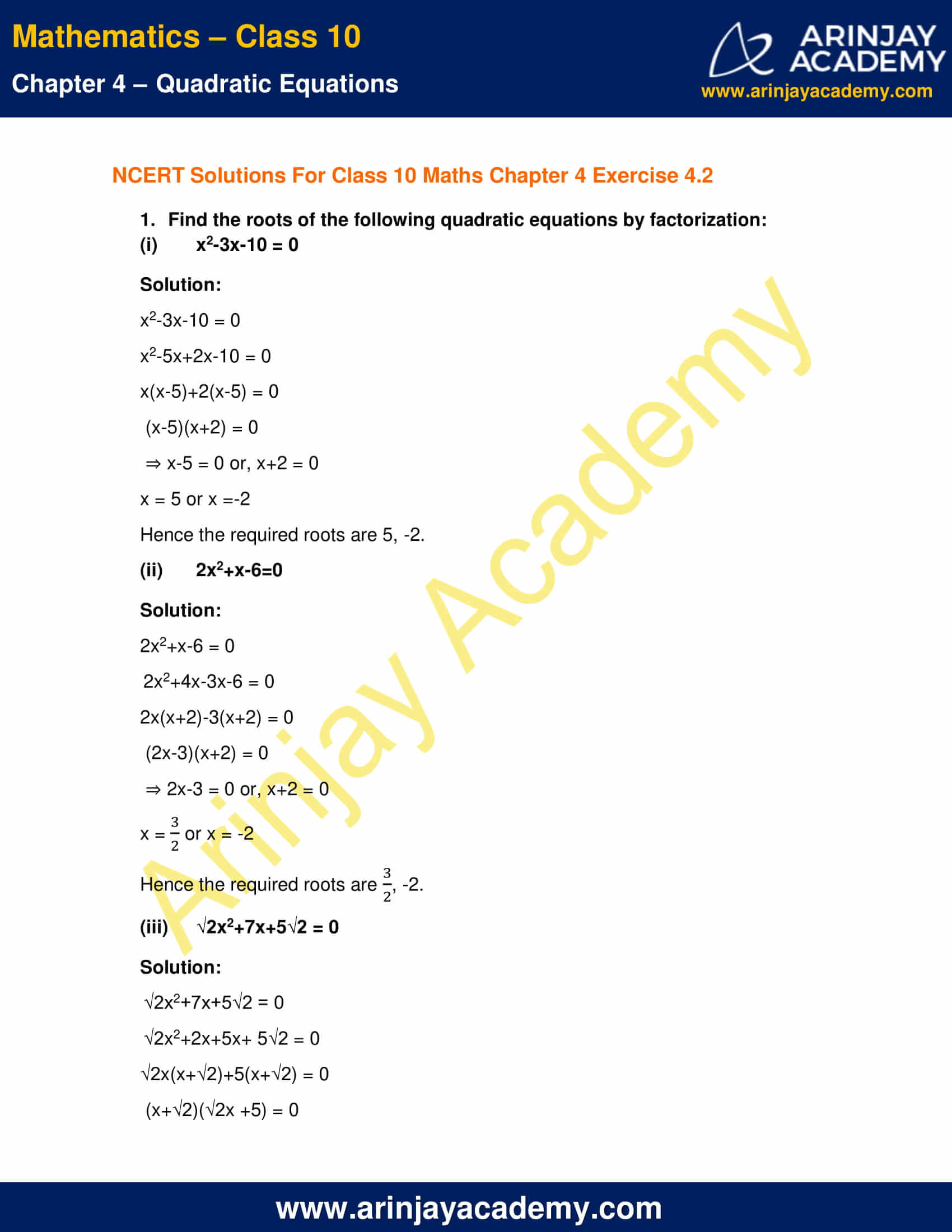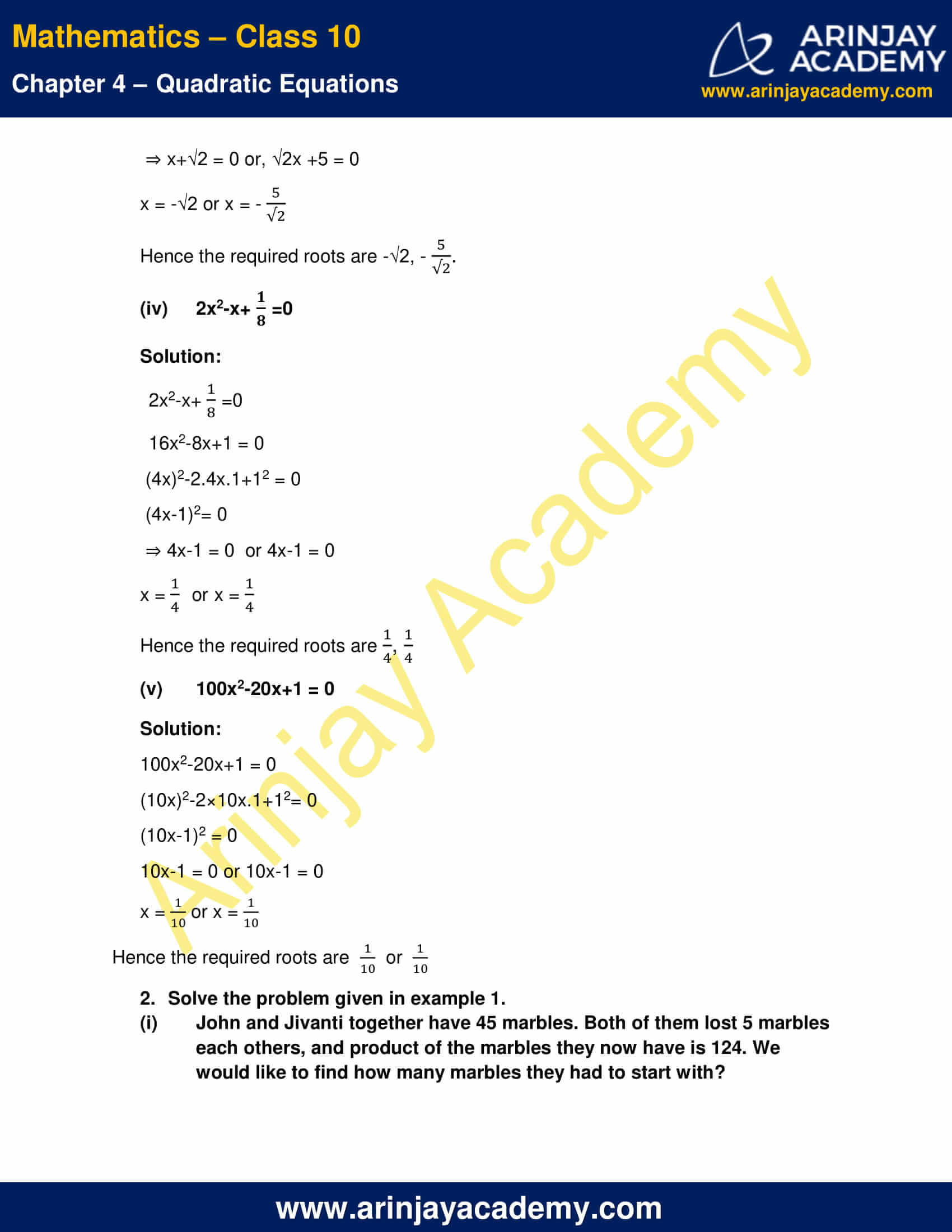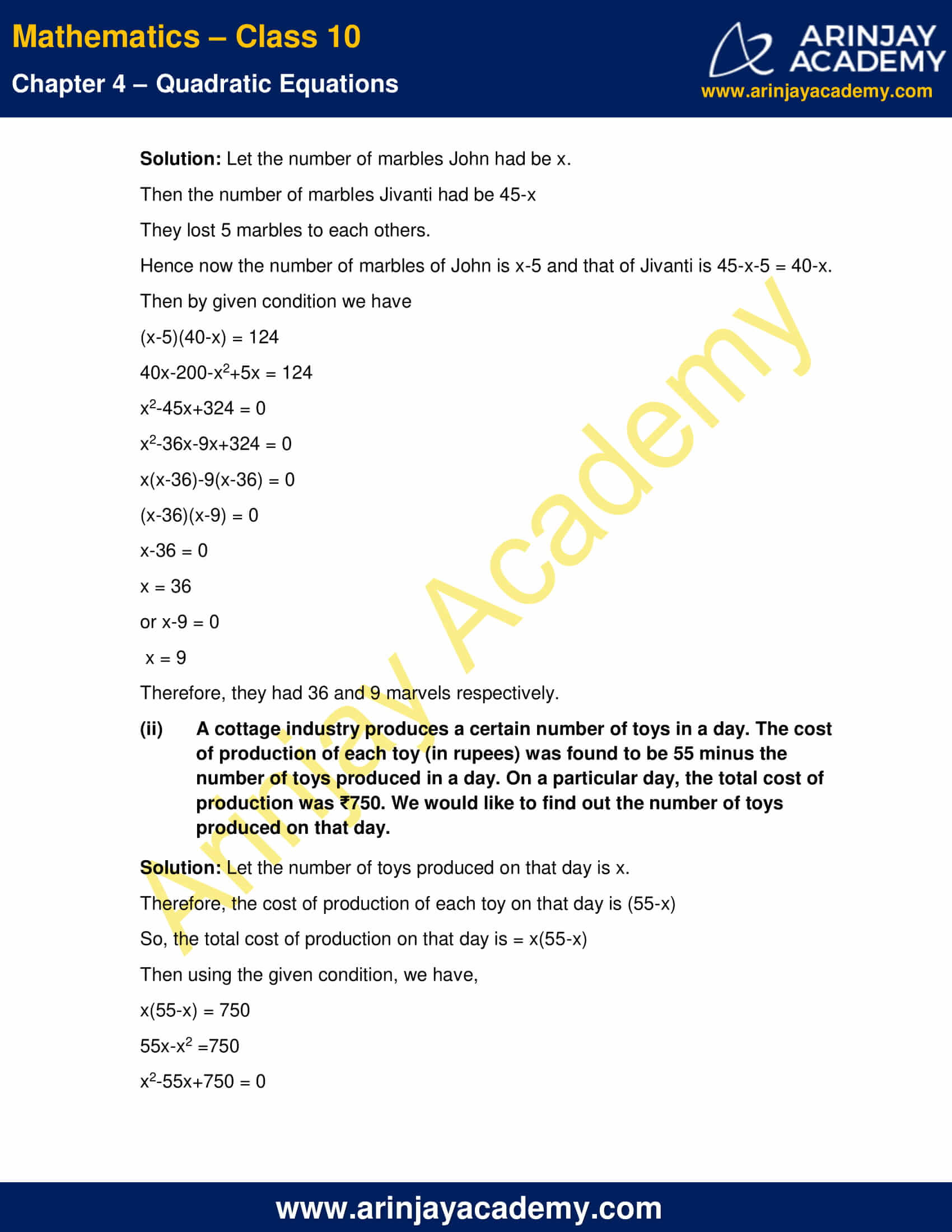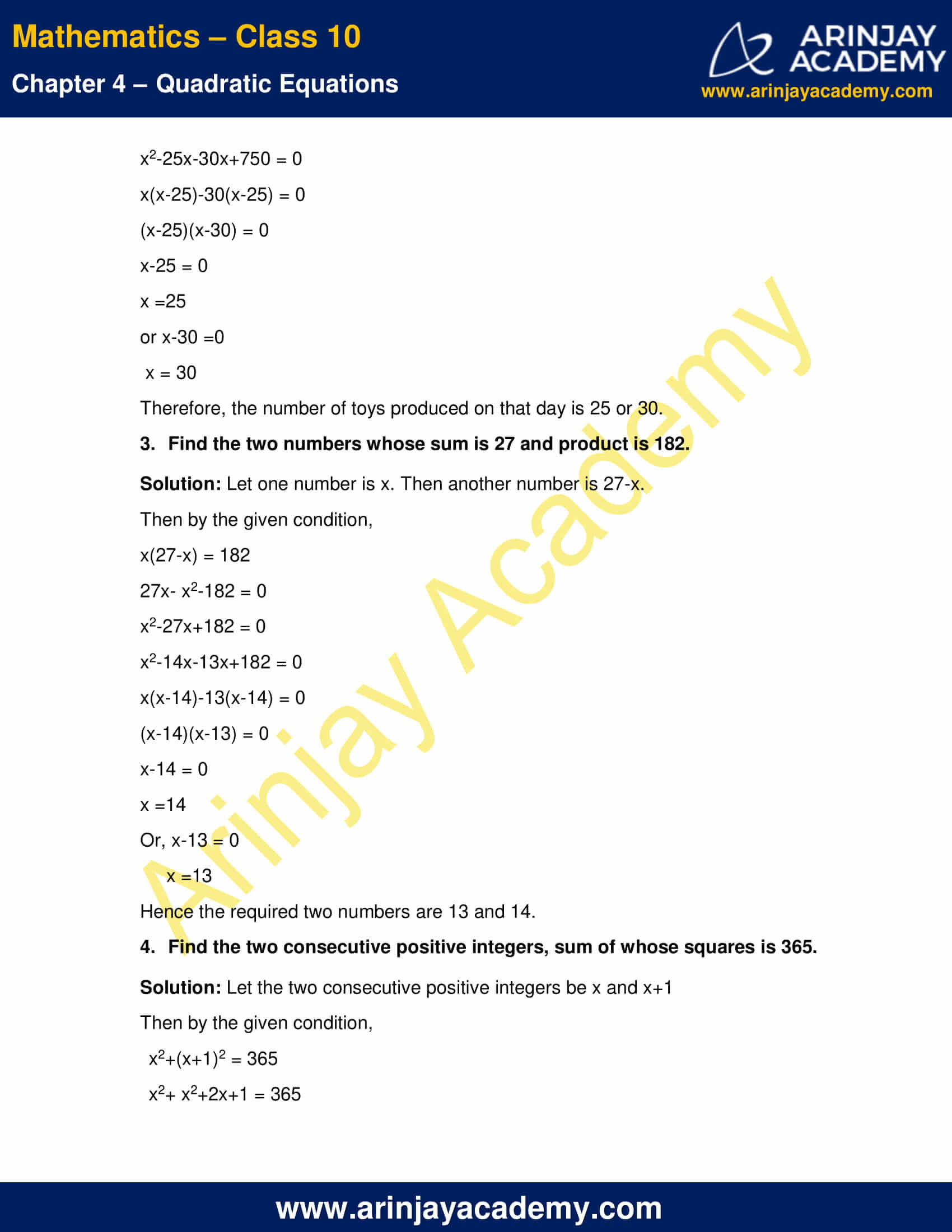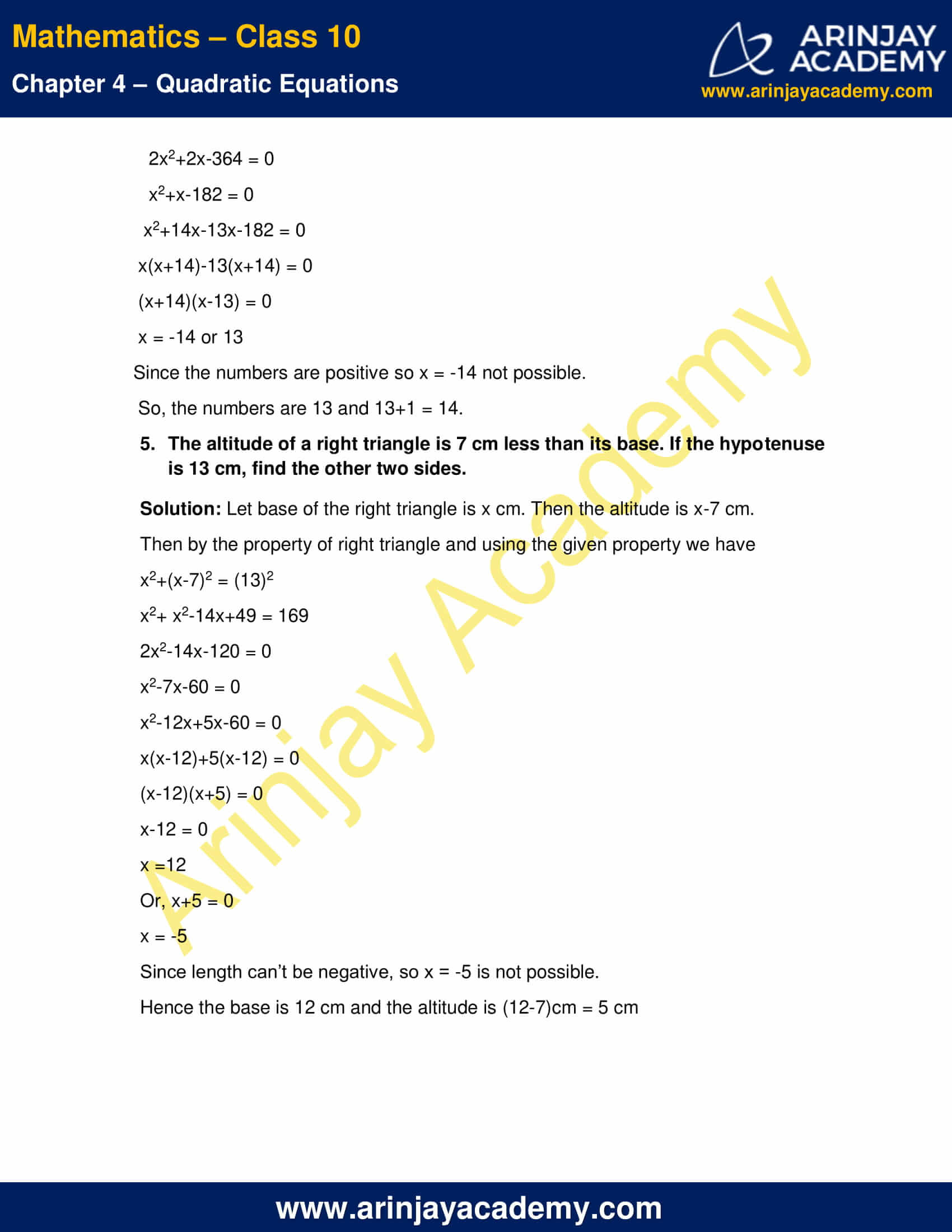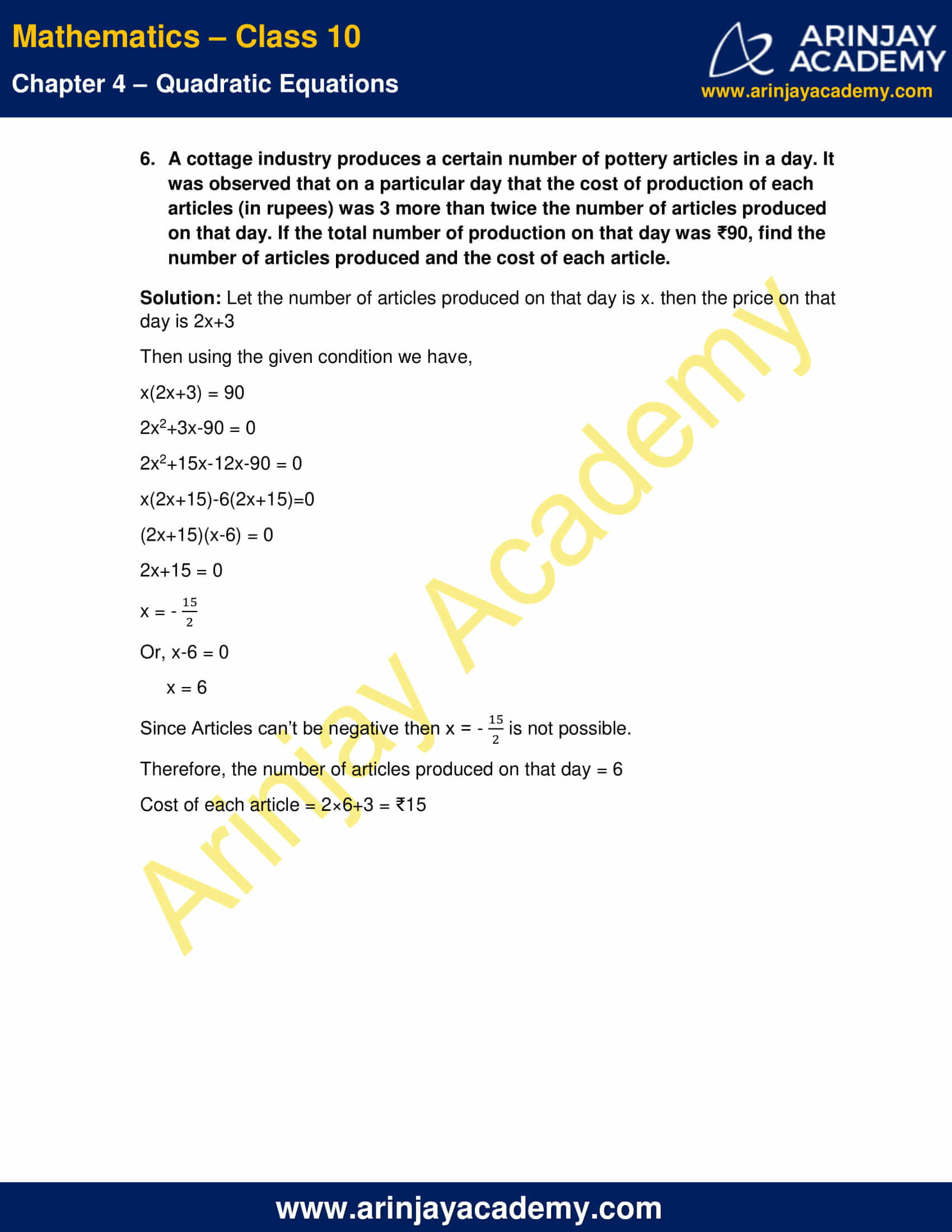NCERT Solutions For Class 10 Maths Chapter 4 Exercise 4.2 – Quadratic Equations

1. Find the roots of the following quadratic equations by factorization:

(i) x2-3x-10 = 0

Solution:

x2-3x-10 = 0
x2-5x+2x-10 = 0
x(x-5)+2(x-5) = 0
(x-5)(x+2) = 0
⇒ x-5 = 0 or, x+2 = 0
x = 5 or x =-2
Hence the required roots are 5, -2.

(ii) 2x2+x-6=0

Solution:

2x2+x-6 = 0
2x2+4x-3x-6 = 0
2x(x+2)-3(x+2) = 0
(2x-3)(x+2) = 0
⇒ 2x-3 = 0 or, x+2 = 0
x = 3/2 or x = -2
Hence the required roots are 3/2, -2.

(iii) √2x2+7x+5√2 = 0

Solution:

√2x2+7x+5√2 = 0
√2x2+2x+5x+ 5√2 = 0
√2x(x+√2)+5(x+√2) = 0
(x+√2)(√2x +5) = 0
⇒ x+√2 = 0 or, √2x +5 = 0
x = -√2 or x = -(5/√2)

Hence the required roots are -√2, -(5/√2).

(iv) 2x2-x+(1/8)  =0

Solution:

2x2-x+ (1/8) =0
16x2-8x+1 = 0
(4x)2-2.4x.1+12 = 0
(4x-1)2= 0
⇒ 4x-1 = 0  or 4x-1 = 0
x = (1/4)  or x = (1/4)
Hence the required roots are 1/4, 1/4

(v) 100x2-20x+1 = 0

Solution:

100x2-20x+1 = 0
(10x)2-2×10x.1+12= 0
(10x-1)2 = 0
10x-1 = 0 or 10x-1 = 0
x = 1/10 or x = 1/10
Hence the required roots are 1/10 or 1/10

2. Solve the problem given in example 1.

(i) John and Jivanti together have 45 marbles. Both of them lost 5 marbles each others, and product of the marbles they now have is 124. We would like to find how many marbles they had to start with?

Solution: Let the number of marbles John had be x.
Then the number of marbles Jivanti had be 45-x
They lost 5 marbles to each others.
Hence now the number of marbles of John is x-5 and that of Jivanti is 45-x-5 = 40-x.
Then by given condition we have
(x-5)(40-x) = 124
40x-200-x2+5x = 124
x2-45x+324 = 0
x2-36x-9x+324 = 0
x(x-36)-9(x-36) = 0
(x-36)(x-9) = 0
x-36 = 0
x = 36
or x-9 = 0
x = 9
Therefore, they had 36 and 9 marvels respectively.

(ii) A cottage industry produces a certain number of toys in a day. The cost of production of each toy (in rupees) was found to be 55 minus the number of toys produced in a day. On a particular day, the total cost of production was ₹750. We would like to find out the number of toys produced on that day.

Solution: Let the number of toys produced on that day is x.
Therefore, the cost of production of each toy on that day is (55-x)
So, the total cost of production on that day is = x(55-x)
Then using the given condition, we have,
x(55-x) = 750
55x-x2 =750
x2-55x+750 = 0
x2-25x-30x+750 = 0
x(x-25)-30(x-25) = 0
(x-25)(x-30) = 0
x-25 = 0
x =25
or x-30 =0
x = 30
Therefore, the number of toys produced on that day is 25 or 30.

3. Find the two numbers whose sum is 27 and product is 182.

Solution: Let one number is x. Then another number is 27-x.
Then by the given condition,
x(27-x) = 182
27x- x2-182 = 0
x2-27x+182 = 0
x2-14x-13x+182 = 0
x(x-14)-13(x-14) = 0
(x-14)(x-13) = 0
x-14 = 0
x =14
or, x-13 = 0
x =13
Hence the required two numbers are 13 and 14.

4. Find the two consecutive positive integers, sum of whose squares is 365.

Solution: Let the two consecutive positive integers be x and x+1
Then by the given condition,
x2+(x+1)2 = 365
x2+ x2+2x+1 = 365
2x2+2x-364 = 0
x2+x-182 = 0
x2+14x-13x-182 = 0
x(x+14)-13(x+14) = 0
(x+14)(x-13) = 0
x = -14 or 13
Since the numbers are positive so x = -14 not possible.
So, the numbers are 13 and 13+1 = 14.

5. The altitude of a right triangle is 7 cm less than its base. If the hypotenuse is 13 cm, find the other two sides.

Solution: Let base of the right triangle is x cm. Then the altitude is x-7 cm.
Then by the property of right triangle and using the given property we have
x2+(x-7)2 = (13)2
x2+ x2-14x+49 = 169
2x2-14x-120 = 0
x2-7x-60 = 0
x2-12x+5x-60 = 0
x(x-12)+5(x-12) = 0
(x-12)(x+5) = 0
x-12 = 0
x =12
or, x+5 = 0
x = -5
Since length can’t be negative, so x = -5 is not possible.
Hence the base is 12 cm and the altitude is (12-7)cm = 5 cm

6. A cottage industry produces a certain number of pottery articles in a day. It was observed that on a particular day that the cost of production of each articles (in rupees) was 3 more than twice the number of articles produced on that day. If the total number of production on that day was ₹90, find the number of articles produced and the cost of each article.

Solution: Let the number of articles produced on that day is x. then the price on that day is 2x+3
Then using the given condition we have,
x(2x+3) = 90
2x2+3x-90 = 0
2x2+15x-12x-90 = 0
x(2x+15)-6(2x+15)=0
(2x+15)(x-6) = 0
2x+15 = 0
x = – (15/2)
or, x-6 = 0
x = 6
Since Articles can’t be negative then x = -(15/2) is not possible.
Therefore, the number of articles produced on that day = 6
Cost of each article = 2×6+3 = ₹15

NCERT Solutions for Class 10 Maths Chapter 4 Exercise 4.2 – Quadratic Equations, has been designed by the NCERT to test the knowledge of the student on the topic – Solution of a Quadratic Equation by Factorisation